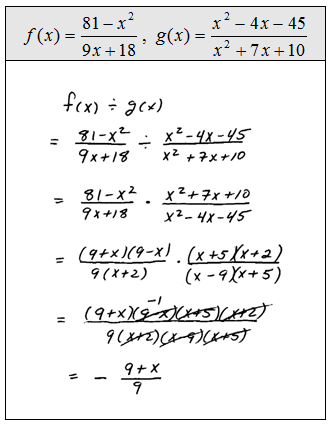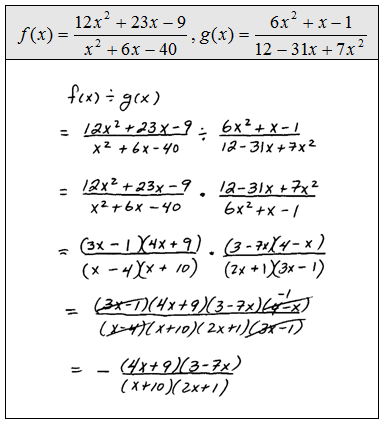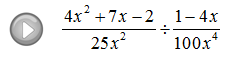## Algebra

Showing posts with label expressions. Show all posts
Showing posts with label expressions. Show all posts

Now when working with square roots and variables we should be a bit careful.

The variable could represent a positive or negative number so we must ensure that it is positive by making use of the absolute value.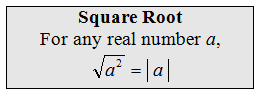To avoid this technicality many textbooks state, at this point, that we assume all variables are positive. If not, use the absolute values as in the following problems.
Simplify. (Assume variables could be negative.)To avoid many of the technicalities when working with nth roots we will assume, from this point on, that all the variables are positive.

Simplify. (Assume all variables represent positive numbers.)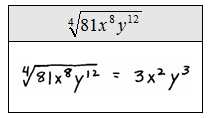Students often try to simplify the previous problem. Be sure to understand what makes the following problems all different.The property: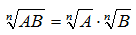says that we can simplify when the operation is multiplication.  There is no corresponding property for addition or subtraction.

### Multiplying and Dividing Rational Expressions

Multiplying rational expressions is similar to simplifying them.  We will factor then cancel.

Only cancel factors that are the same, or opposites, in the numerator and denominator.  Recall the property for multiplying fractions,When multiplying fractions there is no need for a common denominator, just multiply the numerators and denominators and then simplify.

Multiply.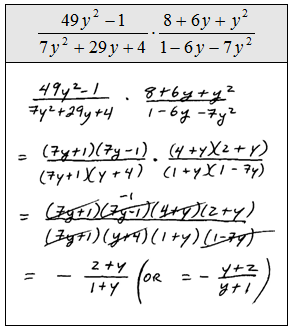The previous questions did not ask for the restrictions but we certainly can list them anyway. Look at the factors in the denominator to see what values for x will evaluate it to zero. Remember that the function notation that implies multiplication.For the given functions find (f *g)(x).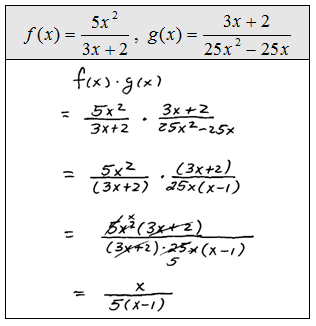When dividing fractions there is no need for a common denominator.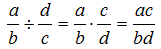However, the above property reminds us that when dividing by a fraction the result will be the same as multiplying by the reciprocal of that fraction. At this point, we will add a step when dividing; we need to reciprocate, factor then cancel.

Divide.The list of restrictions in the previous problem is a bit more involved. As before, look at all the factors in the denominator, even if it was cancelled, to find the values that evaluate to zero.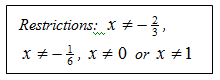Look at the denominators in each step to identify the restrictions.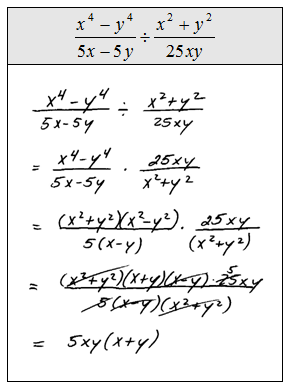Remember that the function notation that implies division.For the given functions find (f / g)(x).